﻿ Calculation of Radiant Section Temperatures in Fired Process Heaters

### Calculation of Radiant Section Temperatures in Fired Process Heaters

Hassan Al-Haj Ibrahim, M. Mourhaf Al-QassimiOPEN ACCESSPEER-REVIEWED

## Calculation of Radiant Section Temperatures in Fired Process Heaters

Hassan Al-Haj Ibrahim1,, M. Mourhaf Al-Qassimi1

1Department of Chemical Engineering, Al-Baath University, Homs, Syria

### Abstract

Flame and effective gas temperatures are key variables that need to be accurately determined before analysis of heat transfer in the radiant section of fired heaters can be meaningfully undertaken. To facilitate the calculation of these temperatures, appropriate equations were derived using two Computer Matlab programmes specially written for the purpose. A third programme was also written for the solution of the derived equations using the Newton-Raphson method. The whole calculation procedure was illustrated by an example worked out for an actual process heater used in a crude oil topping unit.

• Ibrahim, Hassan Al-Haj, and M. Mourhaf Al-Qassimi. "Calculation of Radiant Section Temperatures in Fired Process Heaters." Chemical Engineering and Science 1.4 (2013): 55-61.
• Ibrahim, H. A. , & Al-Qassimi, M. M. (2013). Calculation of Radiant Section Temperatures in Fired Process Heaters. Chemical Engineering and Science, 1(4), 55-61.
• Ibrahim, Hassan Al-Haj, and M. Mourhaf Al-Qassimi. "Calculation of Radiant Section Temperatures in Fired Process Heaters." Chemical Engineering and Science 1, no. 4 (2013): 55-61.

 Import into BibTeX Import into EndNote Import into RefMan Import into RefWorks

### 1. Introduction

Fired process heaters are furnaces in which a process fluid flowing inside tubes located in the furnace is heated by gases produced by the combustion of a liquid or gaseous fuel. Such heaters are widely used in oil refineries and petrochemical plants for heating purposes. They are of two basic types: vertical cylindrical or box-type heaters [1, 2, 3]. For the most part, tubular heaters consist of two main sections: a radiant section, variously called a combustion chamber or firebox, in which heat is transferred mainly by radiation, and a convection section followed by the stack. Roughly-speaking, about 45-55% of the total heat release in the furnace is transferred to the process fluid in the radiant section, leaving about 25-45% of the total heat release to be either transferred to the process fluid in the convection section or carried by the flue gases through the stack and is lost .

### 2. Heat Transfer Mechanisms in Fired Heaters

In the usual practice, the process fluid is first heated in the convection section preheat coil which is followed by further heating in the radiant section. In both sections heat is transferred by both mechanisms of heat transfer, viz. radiation and convection, where radiation is the dominant mode of heat transfer in the radiant section and convection predominates in the convection section as the average temperature in this section is much lower. The heat-absorbing surface in both sections is the outside wall of the tubes mounted inside the heater.

Total heat transfer to the process fluid can be estimated using the following equation: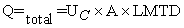(1)

The radiant heat transfer follows the relationship: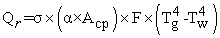(2)

and convective heat transfer follows the relationship: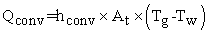(3)

A number of key variables serve as a basis for the determination of heater performance. These include :

1. Inlet and outlet process fluid temperatures.

2. Effective flue gas and flame temperatures.

3. Tube skin or tube wall temperature.

4. Heat transfer rates to the process fluid.

5. Flue gas composition.

6. Process fluid flow rate.

7. Fuel flow rate.

8. Process fluid pressure drop.

9. Pressure profile in the heater and stack.

Of these variables, the effective gas and flame temperatures are most important for a comprehensive heat transfer analysis of the heater radiant section. Furthermore, efficiency calculations require prior calculation of these two temperatures .

### 3. Effective Gas Temperature (Tg)

The effective gas temperature is the temperature controlling radiant transfer in the heater radiant section. For a "well mixed" radiant section this temperature is assumed to be equal to the bridgewall temperature, i.e. the exit temperature of the flue gases leaving the radiant section. For most applications, this is an acceptable assumption with the notable exception of high temperature heaters with tall narrow fireboxes and wall firing where the effective gas temperature may be 95 to 150°C higher than the bridgewall temperature. In this and other cases where the two temperatures differ widely and an adjustment may be necessary, the use of a more accurate gas temperature may have to be considered or the radiant section may have to be divided into zones for the energy balance calculations .

Furthermore, complete flue gas mixing in the firebox is normally assumed in most methods used for the estimation of the effective gas and other radiant section temperatures, including the widely-used Lobo-Evans method . This ignores in effect the existence of longitudinal and transverse temperature gradients. Cross, on the other hand, evaluated the temperature gradients in fired heaters in order to predict the bridgewall temperature .

### 4. Derivation of Effective Gas Temperature Equation

There are two primary sources of heat input to the radiant section, the combustion heat of the fuel Qrls, and the sensible heat of the combustion air Qair, fuel Qfuel and the fuel atomization fluid (for liquid fuel when applicable). Of this heat input some heat is absorbed in the radiant section by the radiant QR and shield tubes Qshld, while the remaining heat is either lost through the casing Qlosses or carried by the flue gas to the convection section Qflue gases. By setting up a heat balance equation for fuel gas the flue gas temperature can then be calculated as follows :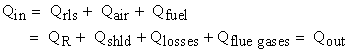(4)

Where: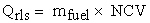(5)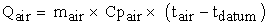(6)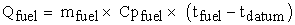(7)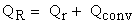(8)

Qr is the radiant heat transfer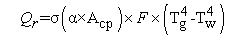(9)

and Q conv is the convective heat transfer in the radiant section.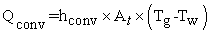(10)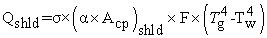(11)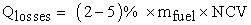(12)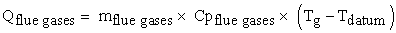(13)

Substitution of the appropriate terms in Equation. 4: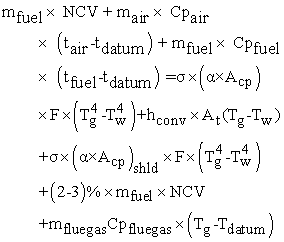(14)

The average tube wall temperature is given by  :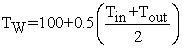(15)

The Newton-Raphson method  was used to solve the heat balance equation and determine the effective gas temperature, for which two Matlab programmes were written (Appendices 1 and 3).

### 5. Flame Temperature (tf)

Flame temperature is the temperature attained by the combustion of a fuel. This temperature depends essentially on the calorific value of the fuel. A theoretical or ideal flame temperature may be calculated assuming complete combustion of the fuel and perfect mixing. But even when complete combustion is assumed, the actual flame temperature would always be lower than the theoretical temperature. There are several reasons for this, chiefly dissociation of the combustion products at higher temperatures and heat loss. Up to a flame temperature of about 1370C, the burned mixture generally includes such ordinary gases as CO2, N2, SO2, H2O and residual O2 (from excess air). At higher temperatures, however, CO2 appreciably dissociates to CO and O2; H2O to O2 and OH-; O2 to O-2; H2 to H+; N2 to N-3; and NO (produced by N2 and O2) to N-3 and O-2. These dissociation reactions absorb an enormous amount of energy, substantially lowering the flame temperature [13, 14]. Further lowering of the flame temperature is also caused by radiation and conduction to the walls of the combustion chamber. Highly turbulent flames usually suffer an appreciable heat loss.

Some work has been done on the calculation of flame temperature, including work by Stehlik and others who studied furnace combustion and drew furnace temperature and enthalpy profiles . Vancini wrote a programme in assembly language for the calculation of the average flame temperature, taking into account dissociation at higher temperatures .

### 6. Derivation of Flame Temperature Equation

In this paper, a simple heat balance serves as the basis for calculating the flame temperature. The increase in enthalpy between the unburned and burned mixtures is assumed to be equal to the heat produced by the combustion. When the fuel is fired, the heat liberated raises the temperature of the combustion products from t1 to t2 so that the following relationship is satisfied: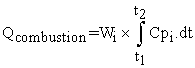(16)

Where:

Qcombustion = Heat of combustion of fuel.

Wi = Mass of a flue gas component.

Cpi = Molar heat of a flue gas component.

t1 and t2 = Initial and final temperatures.

The use of Equation (16) allows the calculation of the flame temperature t by iteration using a programmable calculator. The variation of Cpi with temperature can be approximated by a polynomial, having the obvious advantage of being integrated easily. Using a third-degree polynomial, Cpi can be written as: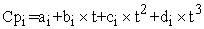(17)

Where, ai, bi, ci and di are constants dependent on the nature of the gas. Assuming t1 to be negligible (= 0), Equation (16) thus becomes: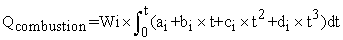(18)

Integrating: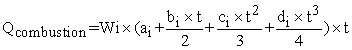(19)

It is customary to call the parenthetic term in Equation (19) the mean molar heat: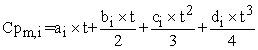(20)

By taking mean molar heats instead of true molar heats, the integration of Equation (16) may be dispensed with. The molar heats at constant pressure for air and flue gases are given in Table 1.

#### Table 1. Molar heats at constant pressure for air and flue gases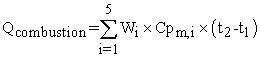(21)

Equation (21) allows the calculation of the theoretical flame temperature, t2, by iteration using a programmable calculator. In order to compensate for the factors that tend to lower the theoretical flame temperature, the heat of combustion is usually multiplied by an empirical coefficient. The values normally used for this coefficient are only estimates; this is why the temperature calculated with any method can only approximate actual values. For an accurate calculation of the actual flame temperature, account must be taken of heat losses through the casing by setting up heat balance equation for fuel gas as follows: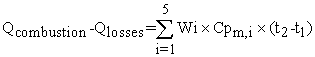(22)

Where: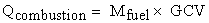(23)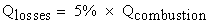(24)

The Newton-Raphson method  was used to solve the heat balance equation and determine the actual flame temperature, for which two Matlab programmes were written (Appendices 2 and 3).

To illustrate the use of the programme, an example is worked out for an actual crude oil heater used in an atmospheric topping unit at the Homs Oil Refinery (Cabin 43-5-16/21 N). In this example, fuel gas is fired with 25% excess air. Ambient temperature = 15°C, exit gas temperature = 400°C. Table 2 gives the furnace characteristics for the radiant section and fuel and combustion air.

The effective gas temperature equation, derived using programme (1), has the following form: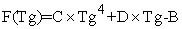(25)

The first derivative of the effective gas temperature equation is: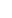(26)

where B, C and D are constants dependent on the type of fuel, percentage of excess air, operating conditions and geometrical characteristics of the fired heater. These constants can then be estimated using Programme 1 as follows (Table 3):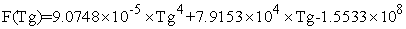(27)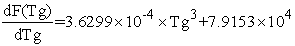(28)

These equations were solved by the Newton-Raphson method in programme (3) to give an effective gas temperature in the fire box equal to 1278K.

The flame temperature equation, derived using programme (2), has the following form: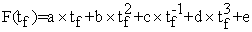(29)

The first derivative of the flame temperature equation is: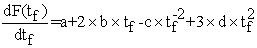(30)

Where a, b, c, d and e are constants estimated dependent on the type of fuel and its gross calorific value, the percentage of excess air and the operating conditions of the fired heater. These constants can then be estimated using Programme 2 as follows (Table 4):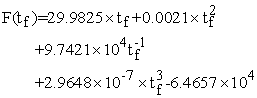(31)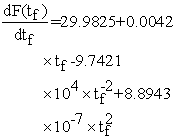(32)

These equations were solved by the Newton-Raphson method using programme (3) to give the actual flame temperature of 2128K.

### 7. Conclusion

Using Matlab programming and the Newton-Raphson method, it was possible to calculate simply and accurately both the effective gas temperature in a fired heater and the actual flame temperature. The calculation was based on heat transfer analysis of the fired heater taking into account heat absorption and losses in the radiant section of the heater. This calculation can be an important tool, not only in the operation and daily running of fired heaters, but also and more importantly for their design.

### Nomenclature

AHeat exchange surface area (m2).

AcpCold plane area of tubes bank in radiation section (m2)

Acp shldCold plane area of shield tubes bank (m2).

AtArea of tubes bank in Radiation section (m2).

CP airMolar heat of combustion air (kJ/kmol.K).

CPfuelSpecific heat of fuel (kJ/kg.deg).

CPflue gasAverage specific heat of flue gases flowing to a bank of bare tubes (kJ/kg.K).

CpiMolar heat of a flue gas component (kJ/kmol.K).

Di, DoInside and outside diameters of tube (mm).

eTube thickness (e = Ro-Ri) (mm)

FExchange factor = 0.97

GCV Gross calorific value of fuel (kJ/h).

hconvFilm convection heat transfer coefficient (kJ/m2.K.h).

LtubeEffective tube length (m)

mairFlow rate of combustion air (kg/h).

mfuelFlow rate of fuel (kg/h).

mflue gasFlow rate of flue gas (kg/h).

NCVNett calorific value of fuel (kJ/h).

n RNumber of tubes in radiation section

N tube (shld)Number of shield tubes.

QairSensible heat of combustion air (kJ/h).

QCTotal heat transfer (kJ/h).

Q combustion Combustion heat of fuel (kJ/h)

Qconv.Convective heat transfer in the radiant section(kJ/h).

Qfuel Sensible heat of fuel (kJ/h).

Qflue gasSensible heat of gas leaving the radiant section(kJ/h)

QRTotal heat transferred to radiant tubes (heat absorbed by radiant tubes) (kJ/h).

QrlsHeat release by burners (kJ/h).

QshldRadiant heat to shield tubes (kJ/h).

Ri, R oInside and outside radii of tube (mm).

Si, SoInside and outside heat surface areas of tube (m2).

StubeTube spacing (m).

t1Temperature of fuel and air (°C)

tfFlame temperature (°C)

TfFlame temperature (K).

TgEffective gas temperature in firebox (K).

TinInlet process fluid temperature (K)

ToutOutlet process fluid temperature ( K)

TwAverage tube-wall temperature (K).

Wi Mass of flue gas component (kmol/h).

### Greek symbols

αRelative effectiveness factor of the tubes bank.

σStefan-Boltzman constant = 2.041×10-7 kJ/h.m2.K4.

### References

  P. Wuithier (ed), Raffinage et génie chimique, L'institut français du pétrole, Paris, 1972, Tome 1.In article  Nelson, W. L., Petroleum Refinery Engineering, 4th ed. McGraw-Hill, New York, 1958.In article  Al-Haj Ibrahim, H., Fired process heaters, Ch. 16, pp. 327-364, in: Matlab, Modelling, programming and simulations, Ed. E. P. Leite, Sciyo, 2010.In article  Stehlik, P., et al., Furnace integration into process justified by detailed calculation using a simple mathematical model, Chemical Engineering and Processing, 34, pp. 9-23, 1995.In article CrossRef  Fired Heaters: A Guide to Performance Evaluation, Prepared by Equipment Testing Procedures Committee, AIChI Equipment Testing Procedure, 1989.In article  Al-Haj Ibrahim, H., Al-Qassimi, M.; Matlab program computes thermal efficiency of fired heater, Periodica Polytechnica, Chemical Engineering, Vol. 52, No. 2, pp. 61-69, 2008.In article  Effective gas temperature in firebox, Available at: www.firedheater.com.In article  Lobo, W. E, Evans, J. E.; Heat Transfer in Radiant Section of Petroleum Heaters, Trans. Am. Inst. Chem. Engrs. 35, pp. 748-778, 1939.In article  Cross, A., Evaluate Temperature Gradients in Fired Heaters, Chemical Engineering Progress, Vol. 98, No.6, pp. 42-46, 2002.In article  Heat Balance in the Radiant Section, Available at: www.firedheater.com.In article  Walas, S. M., Fired heaters, Chemical Process Equipment, Selection and Design, Butterworth-Heinmann, 1990.In article  Chapra Steven C., Applied Numerical Methods with MATLAB for Engineers and Scientists, 1st edition, McGraw-Hill Companies, Inc, 2005.In article  Vacini, C. A., Program calculates flame temperature, Chemical Engineering, pp.133-136, March 22, 1982.In article  Flame Temperature Calculator, Available at: www.firedheater.com.In article  Perry, R. H., Green, D. W.; Perry's Chemical Engineers' Handbook, 8th Edition, McGraw-Hill Publishing, 2008.In article

### Appendix 1

% Program for determination of effective gas temperature.

% Qin=Qrls+Qair+Qfuel

% Input :

ti=input(' Inlet temperature of process fluid (C) =');

Tin=ti+273;

to=input(' Outlet temperature of process fluid (C)= ');

Tout=to+273;

ts=input('Stack Temperature (C)=');

mfuel=input(' Flow rate of fuel (kmol/h)=');

mair=input(' Flow rate of combustion air (kmol/h)=');

mflue=input(' Flow rate of flue gases (kmol/h)=');

N=input (' Number of tubes in radiation section=');

Nshld=input(' Number of shield tube=');

L=input (' Effective tube length(m)=');

Do=input(' External diameter of tube in convection section(m)=');

C=input(' Center-to-Center distance of tube spacing(m)=');

NCV=input('Net Calorific Value of fuel(kJ/kmol)=');

Cpfuel=input(' Molar heat of fuel(kJ/kmol.deg.)=');

% Consrtant :

% Stefan-Boltzman Constant(kJ/h.m^2.K^4)

Sigma=2.041*10^(-7);

F=0.97; % Exchange factor

alpha=0.835; % Relative effectiveness factor of the tubes bank

% Heat input to the radiant section

% Combustion heat of fuel

Qrls=mfuel*NCV;

% Q=mair*Cpair*(tair-tdatum)

tair=25;

tdatum=15;

% Molar heat of air

Cpair=33.915+1.214*10^(-3)*(tair+tdatum)/2;

% Sensible heat of air

Qair=mair*Cpair*(tair-15);

% Qfuel=mfuel*Cpfuel*(tfuel-tdatum)

tfuel=25;

% Sensible heat of fuel

Qfuel=mfuel*Cpfuel*(tfuel-tdatum);

Qin=Qrls+Qair+Qfuel;

% Heat taken out of radiant section

% Qout=QR+Qshld+Qlosses+Qflue

% Heat absorbed by radiant tubes

% QR=Qr+Qconv

% Qr=sigma+(alpha*Acp)*F*(Tg^4-Tw^4)

% Tw = Average tube wall temperature in degrees Kelvin

% Tg = Effective gas temperature in degrees Kelvin

Tw=100+0.5*(Tin+Tout);

% Cold plane area of tube bank

Acp=N*C*L;

% Qconv=hconv*At*(Tg-Tw)

At=N*pi*Do*L; % Area of tubes in bank

hconv=30.66; % Film convective heat transfer coefficient; (kJ/h.m2.c)

% Radiant heat to shield tubes

% Qshld=Nshld*sigma*(alpha*Acp)shld*F*(Tg^4-Tw^4)

% alpha=1, for the shield tubes can be taken to be equal to one.

Acpshld=Nshld*C*L;

% Qshld=Nshld*sigma*(1*Acpshld)*F*(Tg^4-Tw^4)

% Heat losses through setting

Qlosses=0.05*Qrls;

% Qflue=mflue*Cpflue*(Tg-Tdatum)

Tdatum=tdatum+273;

% Molar heat of flue gases

Ts=ts+273;

Cpflue=29.98+3.1157*10^(-3)*(Ts+Tdatum)/2;

% Sensible heat of flue gases

% Qflue=mflue*Cpflue*(Tg-Tdatium)

% Output:

A=Qin-Qlosses;

B=A+Sigma*F*(alpha*Acp+Acpshld)*Tw^4+hconv*At*Tw+mflue*Cpflue*Tdatum;

C=Sigma*F*(alpha*Acp+Acpshld);

D=hconv*At+mflue*Cpflue;

syms Tg

% Equation of effective temperature.

y=C*Tg^4+D*Tg-B;

disp('Equation for effective gas temperature')

func=y

% Finding of first derivative of effective gas temperature equation.

disp('first derivative of effective gas temperature equation')

dfunc=diff(func)

### Appendix 2

% Programme for determination of flame temperature equation.

% Input :

% Flow rate of fuel (kmol/h)

% Flow rate of flue gases (kmol/h)

% Molar Composition of flue gases: XCO2, XH2O, XN2,

% XO2 and XSO2

% Molar heats of flue gases (kJ/kmol.K)

% Percentage of heat losses

% Output:

% Flame temperature (K)

Mfuel=input('Flow rate of fuel(kmol/h)=:');

Mfluegas=input('Flow rate of flue gases(kmol/h)=:');

X=input('percentage of heat losses=:');

GCV=input('Gross calorific value of fuel (kJ/kmol)=:');

XC=input('Molar fraction of CO2=:');

XH=input('Molar fraction of H2O=:');

XN=input('Molar fraction of N2=:');

XO=input('Molar fraction of O2=:');

XS=input('Molar fraction of SO2=:');

td=15; % Datum temperature (C)

% Molar heats at constant pressure for flue gases

% CpCO2=43.2936+0.01147*T-818558.5*T^(-2)

% CpH2O=34.42+6.281*10^(-4)*T+5.611*10^(-6)*T^2

% CpN2=27.2155+4.187*10^(-3)*T

% CpO2=34.63+1.0802*10^(-3)*T-785900*T^(-2)

% CpSO2=32.24+0.0222*T-3.475*10^(-6)*T^(2)

% Heat evolved by fuel during combustion (kJ/h)

Q=GCV*Mfuel;

% Heat losses (kJ/h)

Qloss=X*Q;

Qt=Q-Qloss;

syms tf

CpCO2=43.2936+0.01147*tf-818558.5*tf^(-2);

CpH2O=34.42+6.281*10^(-4)*tf+5.611*10^(-6)*tf^2;

CpN2=27.2155+4.187*10^(-3)*tf;

CpO2=34.63+1.0802*10^(-3)*tf-785900*tf^(-2);

CpSO2=32.24+0.0222*tf-3.475*10^(-6)*tf^(2);

% Integration of mean molar heats

Cpm=int(XC*CpCO2+XH*CpH2O+XN*CpN2+XO*CpO2+XS*CpSO2);

H=Cpm-Qt/Mfluegas;

disp('Equation of actual flame temperature')

func=H

% Finding of first derivative of flame temperature equation.

disp('Finding of first derivative of flame temperature equation')

dfun=diff(func)

### Appendix 3

% Solution of effective gas and flame temperature by

% Newton Raphson method

function root=newtraph(func,dfunc,xr,es,maxit)

% newtraph(func,dfunc,xguess,es,maxit):

% Uses Newton-Raphson method to find a function

% input:

% func=name of function

% dfunc=name of derivative of function

% xguess=initial guess

% es=(optional) stopping maximum allowable iterations

% output:

% root =real root

% if necessary, assign default values

if nargin<5, maxit=50; end % if maxit blank set to 50

if nargin<4, es=0.001; end % if es blank set to 0,001

% Newton-Raphson

iter=0;

while (1)

xrold=xr;

xr=xr-func(xr)/dfunc(xr);

iter=iter+1;

if xr~=0, ea=abs((xr-xrold)/xr)*100; end

if ea<=es|iter>=maxit, break, end

end

root=xr;

if root>1300

root=root+273;

fprintf('The actual flame temperature is %8.0f K\n',root)

else root=xr+273;

fprintf('The Effective gas temperature is%8.0f K\n',root)

end